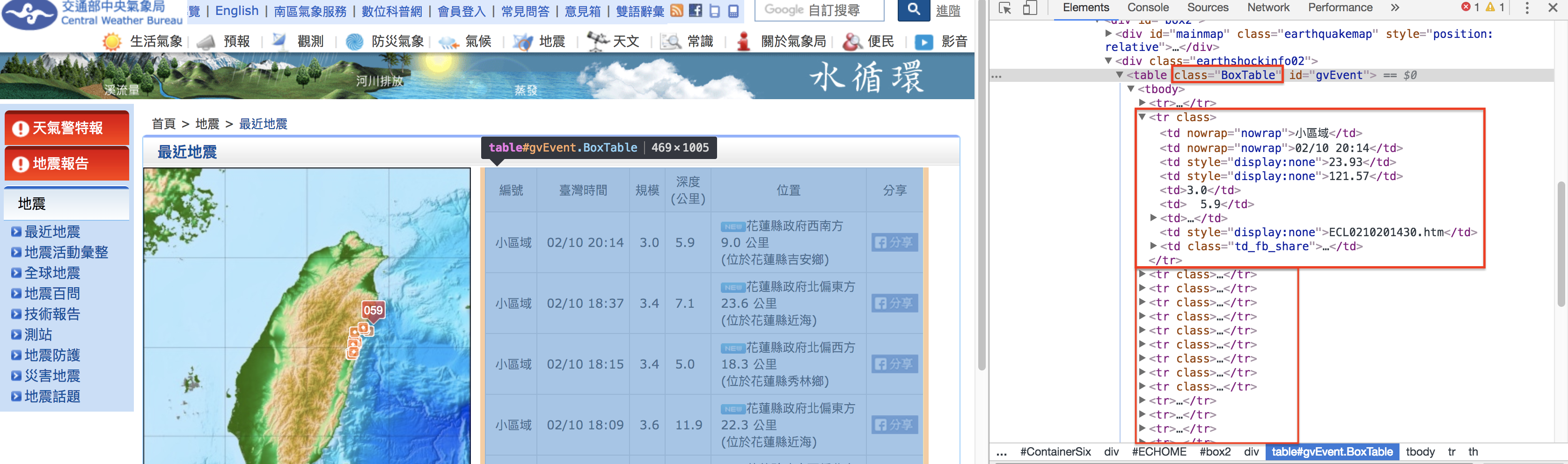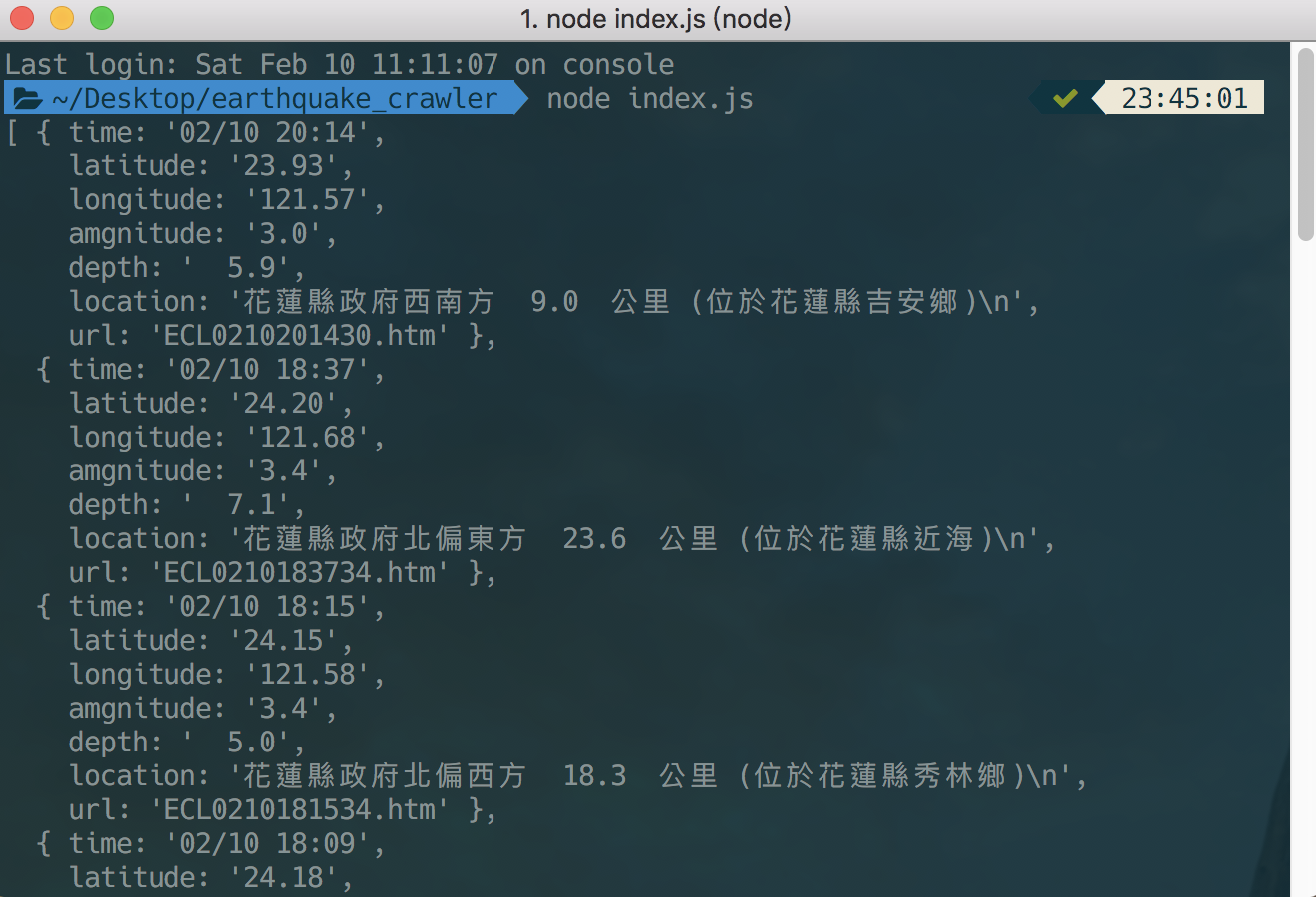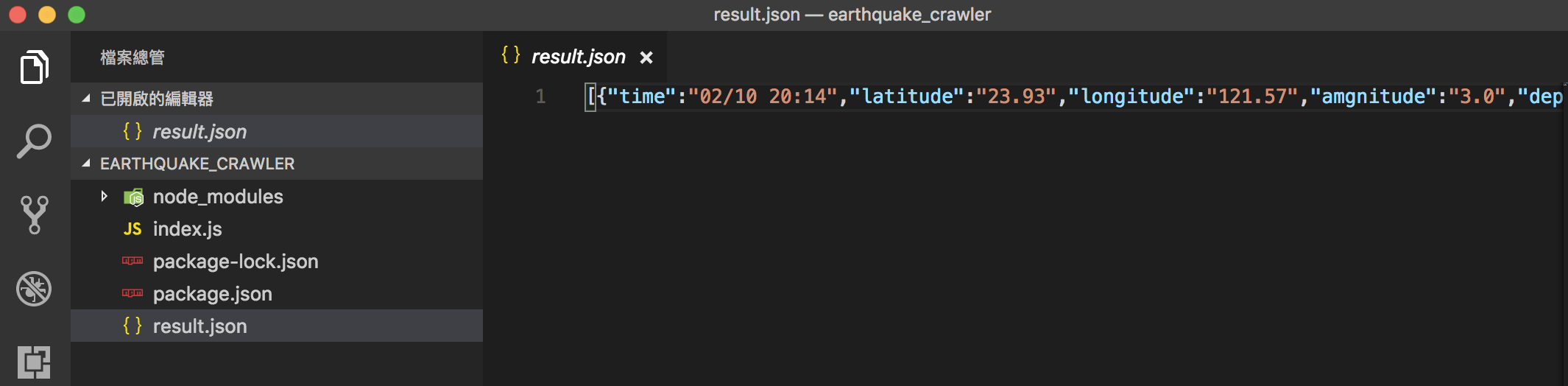# [Node.js 爬蟲] 用 request + cheerio 抓取地震資訊

2018/02/11

## 安裝 request 與 cheerio

``````npm install request cheerio
``````

## 實作``````const request = require("request");
const cheerio = require("cheerio");
const fs = require("fs");

const earthquake = function () {
request({
url: "http://www.cwb.gov.tw/V7/modules/MOD_EC_Home.htm", // 中央氣象局網頁
method: "GET"
}, function (error, response, body) {
if (error || !body) {
return;
}
const \$ = cheerio.load(body); // 載入 body
const result = []; // 建立一個儲存結果的容器
const table_tr = \$(".BoxTable tr"); // 爬最外層的 Table(class=BoxTable) 中的 tr

for (let i = 1; i < table_tr.length; i++) { // 走訪 tr
const table_td = table_tr.eq(i).find('td'); // 擷取每個欄位(td)
const time = table_td.eq(1).text(); // time (台灣時間)
const latitude = table_td.eq(2).text(); // latitude (緯度)
const longitude = table_td.eq(3).text(); // longitude (經度)
const amgnitude = table_td.eq(4).text(); // magnitude (規模)
const depth = table_td.eq(5).text(); // depth (深度)
const location = table_td.eq(6).text(); // location (位置)
const url = table_td.eq(7).text(); // url (網址)
// 建立物件並(push)存入結果
result.push(Object.assign({ time, latitude, longitude, amgnitude, depth, location, url }));
}
// 在終端機(console)列出結果
console.log(result);
// 寫入 result.json 檔案
fs.writeFileSync("result.json", JSON.stringify(result));
});
};

earthquake();
// 每半小時爬一次資料
setInterval(earthquake, 30 * 60 * 1000);
``````https://www.youtube.com/channel/UCSNPCGvMYEV-yIXAVt3FA5A